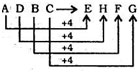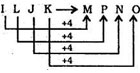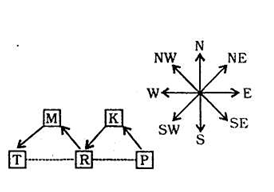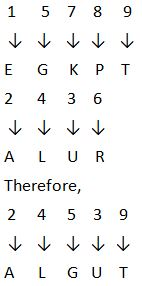# KPMG Reasoning Question

DIRECTIONS for questions 1 – 2: In each of the following questions, select the related letters/word/number from the given alternatives.
1. Patrol: Security : : Insurance : ?
1. Money
2. Policy
3. Savings
4. Risk
In order to ensure security, police or defense personnel patrol the area. Similarly, to cover risk, insurance is done.
2. ADBC : EHFG : : ILJK : ?
1. MOPN
2. MPNO
3. ORPQ
4. MPONSimilarly,1. A and B are standing at a distance of 20 km from each other on a straight East-West road. A and B start walking simultaneously eastwards and westwards respectively and both cover a distance of 5 km. Then A turns to his left and walks 10 km. B turns to his right and walks 10 km at the same speed. Then both turn to their left and cover a distance of 5 km at the same speed. What will be the distance between them?
1. 10 km
2. 30 km
3. 20 km
4. 25 kmRequired distance = 5 + 5 = 10 km
2. From the given alternative words, select the word which cannot be formed using the letters of the given word : REFORMATION'
1. REFRAIN
2. MOTION
3. REFRACT
4. FORMAT
There is no ‘C’ letter in the keyword so REFRACT cannot be formed.
DIRECTIONS for the question 5: In each of the following questions, select the missing number from the given responses.
3.
 169 64 81 30 625 ? 49 50 1296 576 100 70
1. 324
2. 289
3. 441
4. 361
First Row
⇒ √l69+ √64+ √81
= 13 + 8 + 9 = 30
Second Row
⇒ √625 + √? + √49 =50
⇒ 25+ √? +7 = 50
⇒ √? = 50 - 32 = 18
⇒ (?) = (18)2 = 324
Third Row
⇒ √1296 + √576 + √100
= 36 + 24 + 10 = 70
4. Little wooden cubes each with a side of one inch are put together to form a solid cube with a side of three inches. This big cube is then painted red all over on the outside. When the big cube is broken up into the original little ones, how many cubes will have paint on two sides?
1. 4
2. 8
3. 12
4. 0Each edge of the bigger cube will have one small cube with two sides painted. Total number of edges in a cube are 12. Thus 12 is the right option.
5. K is a place which is located 2 km away in the north-west direction from the capital P. R is another place that is located 2 km away in the south-west direction from K. M is another place and that is located 2 km away in the north-west direction from R. T is yet another place that is located 2 km away in the south-west direction from M. In which direction is T located in relation to P?
1. South-west
2. North-west
3. West
4. NorthIt is clear that T is located to the West of P.
6. If in a certain language, PLAYER is coded as QNDCJX, then how SINGER will be coded in the same language?
1. TKQKJX
2. TKJKQX
3. TKQKXJ
4. TKQXJK
The logic followed is that the alphabets in the coded form are increasing by 1, 2, 3….. and so on.
7. If in a certain code, 95789 is written as EGKPT and 2436 is written as ALUR, then how will 24539 be written in that code?
1. ALEUT
2. ALGTU
3. ALGUT
4. ALGRT